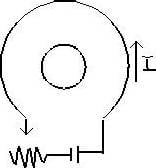# Current, changing B field

meganw

## Homework Statement

(a) If the resistance of the resistor in the figure below is slowly increased, what is the direction of the current induced in the small circular loop inside the larger loop?
(b) What would it be if the small loop were placed outside the larger one, to the left?## Homework Equations

V = IR
E (emf) = d(flux)/dt
flux = B(perp.) * A

## The Attempt at a Solution

I know we have to use the right hand rule, but I think I must be doing it wrong.

For a, we know that B must be decreasing becase I is decreasing (and B = u(o) * I(encl.)/dl)

According to Lentz's law, if the flux(B*A) is decreasing then the induced current will have the same field as the other. So in order for both fields to be pointing out as they are, the current must go in the counterclockwise direction in the inner loop.

for part b though, I use the right hand rule and get the same thing-counterclockwishe? But the answer says its clockwise. I don't understand. The flux of the large loop is still decreasing, so doesn't that mean the fields again point in the same direction? That would be both pointing outward. If I use my RHR that I know, in order for the B field to be pointing in the same direction of the induced current, it must go counterclockwise.

Is there something to be said for the fact that it is outside of the loop?

Thanks!